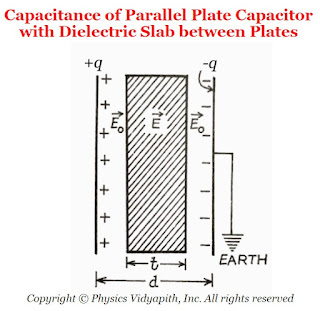### Capacitance of a Parallel Plate Capacitor Partly Filled with Dielectric Slab between Plates

Derivation→ Let us consider,

The charge on a parallel-plate capacitor = $q$
The area of parallel-plate = $A$
The distance between the parallel-plate = $d$
The dielectric constant of the slab of a material =$K$
The thickness of the material =$t$
The vacuum (or air) between the plates =$(d-t)$ The surface charge density on the plates= $\sigma$The capacitance of Parallel Plate Capacitor with Dielectric Slab between Plates
The electric field in the air between the plates is

$E_{\circ}=\frac{\sigma}{\epsilon_{\circ}}$

$E_{\circ}=\frac{q}{\epsilon_{\circ}\: A} \qquad(1)$

The electric field in the dielectric material

$E=\frac{q}{\epsilon_{\circ}\: K\: A} \qquad(2)$

The potential difference between the plates

$V=E_{\circ}\left( d-t \right)+E\:t \qquad(3)$

Now substitute the value of $E_{\circ}$ and $E$ in the equation $(3)$, Then we get

$V=\frac{q}{\epsilon_{\circ}\: A} \left( d-t \right)+ \frac{q}{\epsilon_{\circ}\: K\: A} \:t$

$V=\frac{q}{\epsilon_{\circ}\: A} \left[ \left( d-t \right)+ \frac{t}{K} \right] \qquad$

The capacitance of the capacitor is

$C=\frac{q}{V}$

Now substitute the value of electric potential $V$ in the above equation then

$C= \frac{q}{\frac{q}{\epsilon_{\circ}\: A} \left[ \left( d-t \right)+ \frac{t}{K} \right]}$

 $C= \frac{\epsilon_{\circ}\: A}{\left[ \left( d-t \right)+ \frac{t}{K} \right]}$

Since $K>1$, the 'effective' distance between the plates becomes less than $d$ and so the capacitance increases.

Special Cases→

1. When the dielectric slab is completely filled between the parallel plates i.e. $t=d$ then the capacitance between the parallel plates

 $C= \frac{K \: \epsilon_{\circ}\: A}{d}$

2. When there is a vacuum (or air) between the parallel plates i.e. $t=0$, then the capacitance between the parallel plates

 $C_{\circ}= \frac{K \: \epsilon_{\circ}\: A}{d}$

3. When there is a slab of metal whose dielectric constant is infinity (K=∞). If the thickness of the slab is t between the parallel plates, then the capacitance between the parallel plates

 $C= \frac{ \: \epsilon_{\circ}\: A}{d-t}$

4. When the slabs of dielectric constants $K_{1},K_{2},K_{3},K_{4}...........$ and respective thickness $t_{1},t_{2},t_{3},t_{4},........$ is placed in the entire space between the parallel-plates, then the capacitance between the plates

 $C=\frac{\epsilon_{\circ} A}{d- \left( t_{1}+t_{2}+t_{3}+.... \right)+ \left( \frac{t_{1}}{K_{1}}+\frac{t_{2}}{K_{2}}+\frac{t_{3}}{K_{3}}+....... \right)}$

But $d=t_{1}+t_{2}+t_{3}+....$

 $C=\frac{\epsilon_{\circ} A}{ \left( \frac{t_{1}}{K_{1}}+\frac{t_{2}}{K_{2}}+\frac{t_{3}}{K_{3}}+..... \right)}$